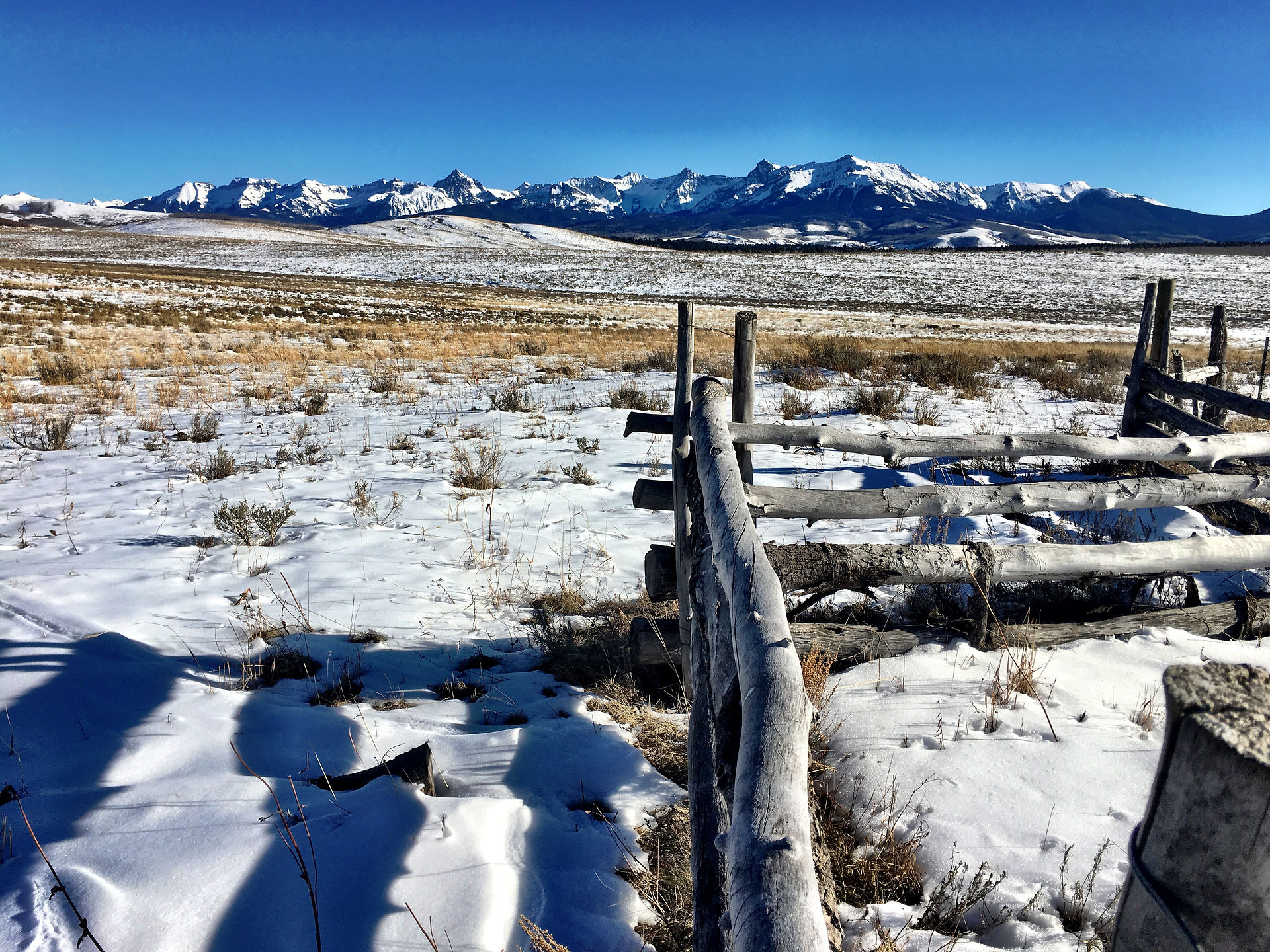top of page`A Rich Bright Blend of Kenya and Malawi AA.`

# African Sunrise

\$18.95Price
• To determine the amount of water to be used with fractional amounts of coffee, multiply the weight of the coffee by the following factors: 16 (0.0625 is the inverse factor) to get fluid ounces of water: 16.6945 (0.0599 is the inverse factor) for grams to get CCs of water.

For example: if you have 1.2 ounces of coffee (by weight), you would multiply 1.2 times 16.0 to get 19.2 fluid ounces of water needed. If you’re using the metric system, 92.6 grams of coffee would require 1562 CCs (1.56 liters) of water. Use the inverse factor to determine the amount of coffee to use with an unlisted amount of water.  In other words, you multiply the inverse factor times the amount of water to determine the weight of the coffee to be used.

bottom of page